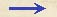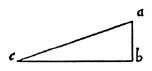Discorsi Propositions 3/02-th-02 Discorsi Proposition3/02-th-02{280} THEOREMA II, PROPOSITIO II. {280} THEOREM II, PROPOSITION IISi aliquod mobile duplici motu aequabili moveatur nempe orizontali et perpendiculari, impetus seu momentum lationis ex utroque motu compositae erit potentia aequalis ambobus momentis priorum motum. When the motion of a body is the resultant of two uniform motions, one horizontal, the other perpendicular, the square of the resultant momentum is equal to the sum of the squares of the two component momenta. Moveatur enim aliquod mobile aequabiliter duplici latione, et mutationi perpendiculari respondeat spatium ab, lationi vero horizontali eodem tempore confectae respondeat bc.Cum igitur per motus aequabiles conficiantur eodem tempore spatia ab, bc, erunt harum lationum momenta inter se ut ipsae ab, bc: mobile vero, quod secundum hasce duas mutationes {10} movetur, describit diagonalem ac; erit momentum suae velocitatis ut ac.Verum ac potentia aequatur ipsis ab, bc; ergo momentum compositum ex utrisque momentis ab, bc est potentia tantum illis simul sumptis aequale: quod erat ostendendum. Let us imagine any body urged by two uniform motions and let ab represent the vertical displacement, while be represents the displacement which, in the same interval a of time, takes place in a horizontal direction. If then the distances ab and bc are traversed, during the same time-interval, with uniform motions (Condition 1/02-th-02) (Condition Impetus-veloc-prop) the corresponding momenta will be to each other as the distances ab and bc are to each other; (Condition 1/02-th-02) (Condition Impetus-veloc-prop) but the body which is urged by these two motions describes the diagonal ac; its momentum is proportional to ac. Also the square of ac is equal to the sum of the squares of ab and bc. Hence the square of the resultant momentum is equal to the sum of the squares of the two momenta ab and bc. Q. E. D.Discorsi Propositions 3/02-th-02 Discorsi Proposition3/02-th-02